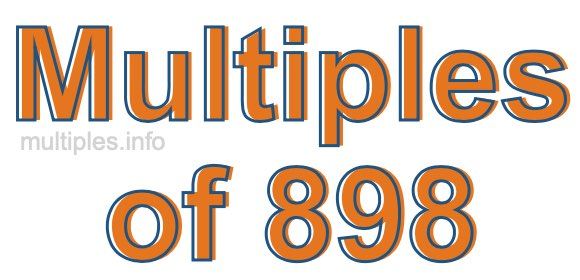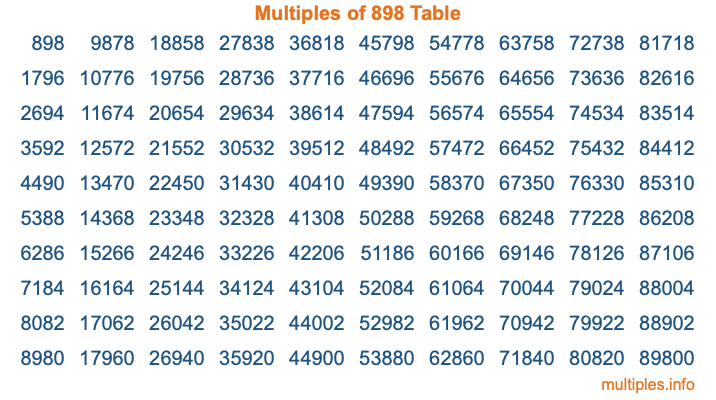Multiples of 898Welcome to the Multiples of 898 page. Here we will first teach you everything you will ever need to know about the multiples of 898, and then give you a study guide summary of everything we taught you to make sure you remember it all. Use this page to look up facts and learn information about the multiples of 898. This page will make you a multiples of eight hundred ninety-eight expert!

Definition of Multiples of 898
Multiples of 898 are all the numbers that when divided by 898 equal an integer. Each of the multiples of 898 are called a multiple. A multiple of 898 is created by multiplying 898 by an integer.

Therefore, to create a list of multiples of 898, you start with 1 multiplied by 898, then 2 multiplied by 898, then 3 multiplied by 898, and so on for as long as you want. Thus, the list of the first five multiples of 898 is 898, 1796, 2694, 3592, and 4490. To see a larger list of multiples of 898, see the printable image of Multiples of 898 further down on this page. We also have a category where you can choose any nth multiple of 898.

Multiples of 898 Checker
The Multiples of 898 Checker below checks to see if any number of your choice is a multiple of 898. In other words, it checks to see if there is any number (integer) that when multiplied by 898 will equal your number. To do that, we divide your number by 898. If the the quotient is an integer, then your number is a multiple of 898.

Is  a multiple of 898?

Least Common Multiple of 898 and ...
A Least Common Multiple (LCM) is the lowest multiple that two or more numbers have in common. This is also called the smallest common multiple or lowest common multiple and is useful to know when you are adding our subtracting fractions. Enter one or more numbers below (898 is already entered) to find the LCM.

Check out our LCM Calculator if you need more details about the Least Common Multiple or if you need the LCM for different numbers for adding and subtraction fractions.

nth Multiple of 898
As we stated above, 898 is the first multiple of 898, 1796 is the second multiple of 898, 2694 is the third multiple of 898, and so on. Enter a number below to find the nth multiple of 898.

th multiple of 898

Multiples of 898 vs Factors of 898
898 is a multiple of 898 and a factor of 898, but that is where the similarities end. All postive multiples of 898 are 898 or greater than 898. All positive factors of 898 are 898 or less than 898.

Below is the beginning list of multiples of 898 and the factors of 898 so you can compare:

Multiples of 898: 898, 1796, 2694, 3592, 4490, etc.

Factors of 898: 1, 2, 449, 898

As you can see, the multiples of 898 are all the numbers that you can divide by 898 to get a whole number. The factors of 898, on the other hand, are all the whole numbers that you can multiply by another whole number to get 898.

It's also interesting to note that if a number (x) is a factor of 898, then 898 will also be a multiple of that number (x).

Multiples of 898 vs Divisors of 898
The divisors of 898 are all the integers that 898 can be divided by evenly. Below is a list of the divisors of 898.

Divisors of 898: 1, 2, 449, 898

The interesting thing to note here is that if you take any multiple of 898 and divide it by a divisor of 898, you will see that the quotient is an integer.

Multiples of 898 Table
Below is an image of the first 100 multiples of 898 in a table. The table is in chronological order, column by column. The first column has the first ten multiples of 898, the second column has the next ten multiples of 898, and so on.The Multiples of 898 Table is also referred to as the 898 Times Table or Times Table of 898. You are welcome to print out our table for your studies.

Negative Multiples of 898
Although not often discussed or needed in math, it is worth mentioning that you can make a list of negative multiples of 898 by multiplying 898 by -1, then by -2, then by -3, and so on, to get the following list of negative multiples of 898:

-898, -1796, -2694, -3592, -4490, etc.

Multiples of 898 Summary
Below is a summary of important Multiples of 898 facts that we have discussed on this page. To retain the knowledge on this page, we recommend that you read through the summary and explain to yourself or a study partner why they hold true.

There are an infinite number of multiples of 898.

A multiple of 898 divided by 898 will equal a whole number.

898 divided by a factor of 898 equals a divisor of 898.

The nth multiple of 898 is n times 898.

The largest factor of 898 is equal to the first positive multiple of 898.

898 is a multiple of every factor of 898.

898 is a multiple of 898.

A multiple of 898 divided by a divisor of 898 equals an integer.

898 divided by a divisor of 898 equals a factor of 898.

Any integer times 898 will equal a multiple of 898.

Multiples of a Number
Here you can get the multiples of another number, all with the same attention to detail as we did for multiples of 898 on this page.

Multiples of
Multiples of 899
Did you find our page about multiples of eight hundred ninety-eight educational? Do you want more knowledge? Check out the multiples of the next number on our list!